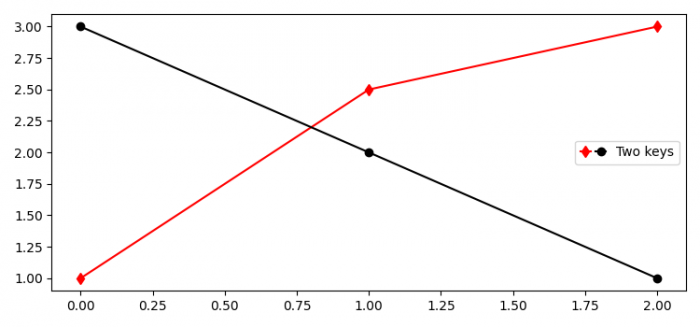# How to make several legend keys to the same entry in Matplotlib?

MatplotlibPythonData Visualization

To make several legend keys to the same entry in Matplotlib, we can take the following steps −

• Set the figure size and adjust the padding between and around the subplots.
• Plot line1 and line2 using plot() method.
• Use legend() method to place a legend over the plot with numpoints=1
• To display the figure, use show() method.

## Example

import matplotlib.pyplot as plt
from matplotlib.legend_handler import HandlerTuple

plt.rcParams["figure.figsize"] = [7.50, 3.50]
plt.rcParams["figure.autolayout"] = True

p1, = plt.plot([1, 2.5, 3], 'r-d')
p2, = plt.plot([3, 2, 1], 'k-o')
l = plt.legend([(p1, p2)], ['Two keys'], numpoints=1, handler_map={tuple: andlerTuple(ndivide=None)})

plt.show()

## Output